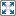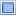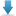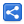# Java 8 Streams - Stateful vs Stateless behavioral parameters

[Last Updated: Feb 7, 2017]

A stateful operation is the one whose result depends on any state that might change during the execution of the pipeline. Stateless operations retain no state during the execution of the pipeline.

## Stateful intermediate operations

Java 8 stream API provides various intermediate operations which are stateful by definition. They maintain some state internally to accomplish the operation.

Those intermediate operations are distinct(), sorted(), limit(), skip(). All other operations are stateless.

These stateful intermediate operations incorporate state from previously processed elements when processing the current element.

## Stateful behavioral parameter

A developer can choose to access/update an external state from a behavioral parameter.

A behavioral parameter is nothing but a functional interface or lambda expression used in intermediate operations.

The problem with stateful behavioral parameter is that: it may render the result nondeterministic, specially for parallel pipeline.

A nondeterministic operation produces different results for the same given input if executed multiple times.

Also accessing external state is not thread-safe and needs to be synchronized.

From Stream API specs:

Note also that attempting to access mutable state from behavioral parameters presents you with a bad choice with respect to safety and performance; if you do not synchronize access to that state, you have a data race and therefore your code is broken, but if you do synchronize access to that state, you risk having contention undermine the parallelism you are seeking to benefit from. The best approach is to avoid stateful behavioral parameters to stream operations entirely; there is usually a way to restructure the stream pipeline to avoid statefulness.

## Examples

Following example shows the usage of stateful behavioral parameter. The pipeline finds the sum of the integers for distinct elements.

The whole process is repeated in a for loop multiple times to see how the results can be non-deterministic.

```public class StatefulExample {

public static void main (String[] args) {
for (int i = 0; i < 5; i++) {

Set<Integer> seen = new HashSet<>();
IntStream stream = IntStream.of(1, 2, 1, 2, 3, 4, 4, 5);
int sum = stream.parallel().map(
//stateful behavioral parameter.
e -> {
return e;
else
return 0;
}).sum();

System.out.println(sum);

}
}
}
```

Output

```19
17
15
17
15
```

Above example can be fixed by using synchronized set, e.g. the one created with
`Collections.synchronizedSet(new HashSet())`
but that would undermine the benefit of parallelism.

We can always find a way to avoid stateful behavioral parameter. Above example can be fixed by replacing stateful lambda expression with distinct operation (which is internally stateful but is safe and deterministic)

```public class StatefulFixExample {

public static void main (String[] args) {
for (int i = 0; i < 5; i++) {
IntStream stream = IntStream.of(1, 2, 1, 2, 3, 4, 4, 5);
int sum = stream.parallel().distinct().sum();
System.out.println(sum);
}
}
}
```

Output

```15
15
15
15
15
```

Here's another example which finds the sum of even numbers and at the same time attempts to find the count of those even numbers by using an external count variable.

```public class StatefulExample2 {
private static int count = 0;

public static void main (String[] args) {
for (int i = 0; i < 5; i++) {
process();
}
}

private static void process () {
count = 0;

IntStream stream = IntStream.range(1, 1000);
//finding the sum of even numbers
int sum = stream.parallel()
.filter(i -> {
boolean b = i % 2 == 0;
if (b) {
count++;//updating count hence making lambda stateful.
}
return b;
})
.sum();

System.out.printf("sum :%d  count:%d%n", sum, count);
}
}
```

Output

```sum :249500  count:365
sum :249500  count:433
sum :249500  count:413
sum :249500  count:437
sum :249500  count:466
```

As seen in the output 'count' is not deterministic.

To fix above code, we are going to find the sum and count separately:

```public class StatefulFixExample2 {
public static void main (String[] args) {
for (int i = 0; i < 5; i++) {
process();
}
}

private static void process () {
IntStream stream = IntStream.range(1, 1000);

//finding the even numbers
int[] even = stream.parallel()
.filter(i -> i % 2 == 0)
.toArray();

//finding sum
int sum = IntStream.of(even).parallel().sum();

System.out.printf("sum :%d  count:%d%n", sum, even.length);
}
}
```

Output

```sum :249500  count:499
sum :249500  count:499
sum :249500  count:499
sum :249500  count:499
sum :249500  count:499
```

Dependencies and Technologies Used:

• JDK 1.8
• Maven 3.0.4

 Stateful Vs Stateless ExampleSelect AllDownload• stateful-behavioral-parameter
• src
• main
• java
• com
• logicbig
• example
• StatefulExample.java

 Share